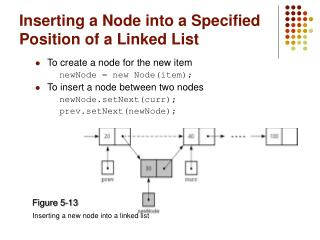# Inserting a Node into a Specified Position of a Linked List - PowerPoint PPT PresentationDownload PresentationInserting a Node into a Specified Position of a Linked List

Inserting a Node into a Specified Position of a Linked ListDownload Presentation## Inserting a Node into a Specified Position of a Linked List

- - - - - - - - - - - - - - - - - - - - - - - - - - - E N D - - - - - - - - - - - - - - - - - - - - - - - - - - -
##### Presentation Transcript

1. Inserting a Node into a Specified Position of a Linked List • To create a node for the new item newNode = new Node(item); • To insert a node between two nodes newNode.setNext(curr); prev.setNext(newNode); Figure 5-13 Inserting a new node into a linked list

2. Inserting a Node into a Specified Position of a Linked List • To insert a node at the beginning of a linked list newNode.setNext(head); head = newNode; Figure 5-14 Inserting at the beginning of a linked list

3. Inserting a Node into a Specified Position of a Linked List • Inserting at the end of a linked list is not a special case if curr is null newNode.setNext(curr); prev.setNext(newNode); Figure 5-15 Inserting at the end of a linked list

4. Inserting a Node into a Specified Position of a Linked List • Three steps to insert a new node into a linked list • Determine the point of insertion • Create a new node and store the new data in it • Connect the new node to the linked list by changing references

5. Determining curr and prev • Determining the point of insertion or deletion for a sorted linked list of objects • assume we have newValue which should inserted (deleted) for ( prev = null, curr = head; (curr != null) && (newValue.compareTo(curr.getItem()) > 0); prev = curr, curr = curr.getNext() ) { } // end for

6. A Reference-Based Implementation of the ADT List • A reference-based implementation of the ADT list • Does not shift items during insertions and deletions • Does not impose a fixed maximum length on the list Figure 5-18 A reference-based implementation of the ADT list

7. A Reference-Based Implementation of the ADT List • Default constructor • Initializes the data fields numItems and head • List operations • Public methods • isEmpty • size • add • remove • get • removeAll • Private method • find

8. Reference-based Implementation of ADT List publicboolean isEmpty() { return numItems == 0; } // end isEmpty publicint size() { return numItems; } // end size

9. Reference-based Implementation of ADT List private Node find(int index) { // -------------------------------------------------- // Locates a specified node in a linked list. // Precondition: index is the number of the desired // node. Assumes that 1 <= index <= numItems+1 // Postcondition: Returns a reference to the desired // node. // -------------------------------------------------- Node curr = head; for (int skip = 1; skip < index; skip++) { curr = curr.getNext(); } // end for return curr; } // end find

10. Reference-based Implementation of ADT List public Object get(int index) throws ListIndexOutOfBoundsException { if (index >= 1 && index <= numItems) { // get reference to node, then data in node Node curr = find(index); Object dataItem = curr.getItem(); return dataItem; } else { thrownew ListIndexOutOfBoundsException( "List index out of bounds on get"); } // end if } // end get

11. Reference-based Implementation of ADT List publicvoid add(int index, Object item) throws ListIndexOutOfBoundsException { if (index >= 1 && index <= numItems+1) { if (index == 1) { // insert the new node containing item at // beginning of list Node newNode = new Node(item, head); head = newNode; } else { Node prev = find(index-1); // insert the new node containing item after // the node that prev references Node newNode = new Node(item, prev.getNext()); prev.setNext(newNode); } // end if numItems++; } else { thrownew ListIndexOutOfBoundsException( "List index out of bounds on add"); } // end if } // end add

12. Reference-based Implementation of ADT List publicvoid remove(int index) throws ListIndexOutOfBoundsException { if (index >= 1 && index <= numItems) { if (index == 1) { // delete the first node from the list head = head.getNext(); } else { Node prev = find(index-1); // delete the node after the node that prev // references, save reference to node Node curr = prev.getNext(); prev.setNext(curr.getNext()); } // end if numItems--; } // end if else { thrownew ListIndexOutOfBoundsException( "List index out of bounds on remove"); } // end if } // end remove

13. Comparing Array-Based and Referenced-Based Implementations • Size (number of elements stored) • Array-based • Fixed size • Can you predict the maximum number of items in the ADT? • Will an array waste storage? • Resizable array • Increasing the size of a resizable array can waste storage and time • Reference-based • Do not have a fixed size • Do not need to predict the maximum size of the list • Will not waste storage (for data)

14. Comparing Array-Based and Referenced-Based Implementations • Storage requirements • Array-based • Requires less memory than a reference-based implementation • There is no need to store explicitly information about where to find the next data item • Reference-based • Requires more storage • An item explicitly references the next item in the list

15. Comparing Array-Based and Referenced-Based Implementations • Access time • Array-based • Constant access time • Reference-based • The time to access the ith node depends on I • But when processing all elements: both implementations require constant time on average

16. Comparing Array-Based and Referenced-Based Implementations • Insertion and deletions • Array-based • Require you to shift the data • Reference-based • Do not require you to shift the data • Require a list traversal